# ASHRAE Calculations

ASHRAE Fundamentals Handbook has a methodology for calculating the friction loss in ducts and fittings. Based on these formulas, Dryer-Ells can be measured the same as straight pipe. This permits use of the maximum allowed run length.

• A ten inch radius smooth interior ninety degree bend will only have an equivalent vent length of 1-1/2 feet (the same length as model LT90).
• A ten inch radius smooth interior forty-five degree bend will only have an equivalent vent length of 3/4 feet (the same length as model LT45).

• ASHRAE Formula as Confirmed JB Engineering and Code Consulting, P.C.: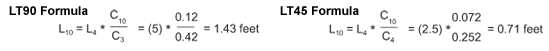Calculation Detail (Using Model LT90 As an Example)
The pressure loss due to friction in a round duct is calculated using the following equation: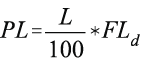Where:PL = Pressure Loss in Duct in Inches of Water Column
L = Length of Duct in Feet
FL = Friction Loss per 100 feet of Duct at Specified Velocity of Flow

The pressure loss through a duct fitting is calculated using the following equation: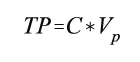Where:TP = Total Pressure Loss in Inches of WC
C = Fitting Coefficient
Vp = Velocity Pressure at Upstream Connection in Inches of WC

The pressure loss through a fitting is often calculated by establishing the equivalent length of duct for each fitting. By using this method of calculating duct pressure loss, the equivalent length of each fitting is added to the total duct length to establish the pressure loss through the duct system.

To establish the equivalent length for a given fitting, the fitting equation is set as being equal to the duct length equation. Solving for “L” establishes the equivalent duct length for a specific fitting with a given velocity of flow through the duct. The equation becomes: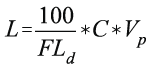A standard three section, 4 inch dryer exhaust duct 90° elbow with a 4 inch radius has been established as having an equivalent length of 5 feet in Section 504.6.1 of the 2003 ICC International Mechanical Code and Section M1501.3 of the ICC International Residential Code. The coefficient for a three section elbow in SMACNA Duct Design Handbook is 0.42.

The coefficient for a 4 inch smooth 90° elbow with a 10 inch radius is 0.12. To establish the equivalent length of dryer exhaust duct for the 4 inch smooth 90° elbow with a 10 inch radius, you cancel out the equivalent factors and are left with the following equation: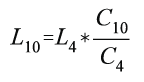Where:L10 = Equivalent length for the 10 inch radius smooth elbow
L4 = Equivalent length for the 4 inch radius, three section elbow
C10 = Coefficient for the 10 inch radius smooth elbow
C4 = Coefficient for the 4 inch radius, three section elbow

Solving the equation results in: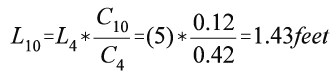Rounding up, the 4 inch smooth 90° elbow with a 10 inch radius would have an equivalent length of dryer vent of 1-1/2 feet.